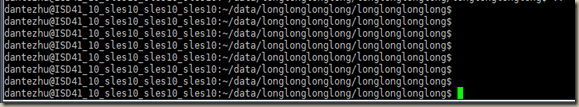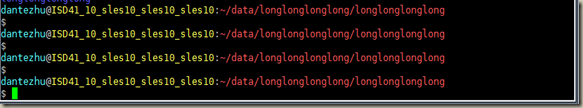# 标签归档：encode

## 【总结】美化bash,python的soap client,python获取系统编码函数``export PS1="\[\e[36;1m\]\u\[\e[0m\]@\[\e[33;1m\]\h\[\e[0m\]:\[\e[31;1m\]\w\[\e[0m\]\n\\$ "``Unfortunately, at the moment, I don't think there is ...

## vim(gvim)一键执行python脚本的bug

``````function CheckPythonSyntax()
let mp = &makeprg
let ef = &errorformat
let exeFile = expand("%:t")
setlocal makeprg=python\ -u
set efm=%C\ %.%#,%A\ \ File\ \"%f\"\\,\ line\ %l%.%#,%Z%[%^\ ]%\\@=%m
silent make %
copen
let &makeprg     = mp
let &errorformat = ef
endfunction``````